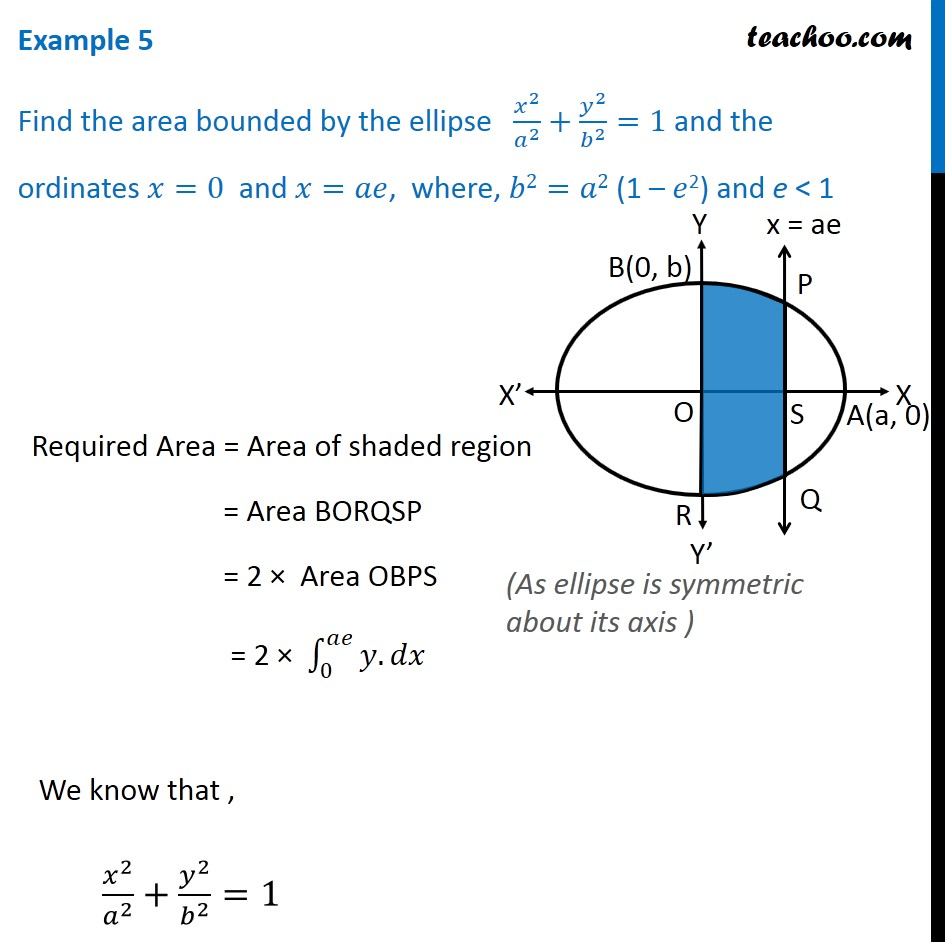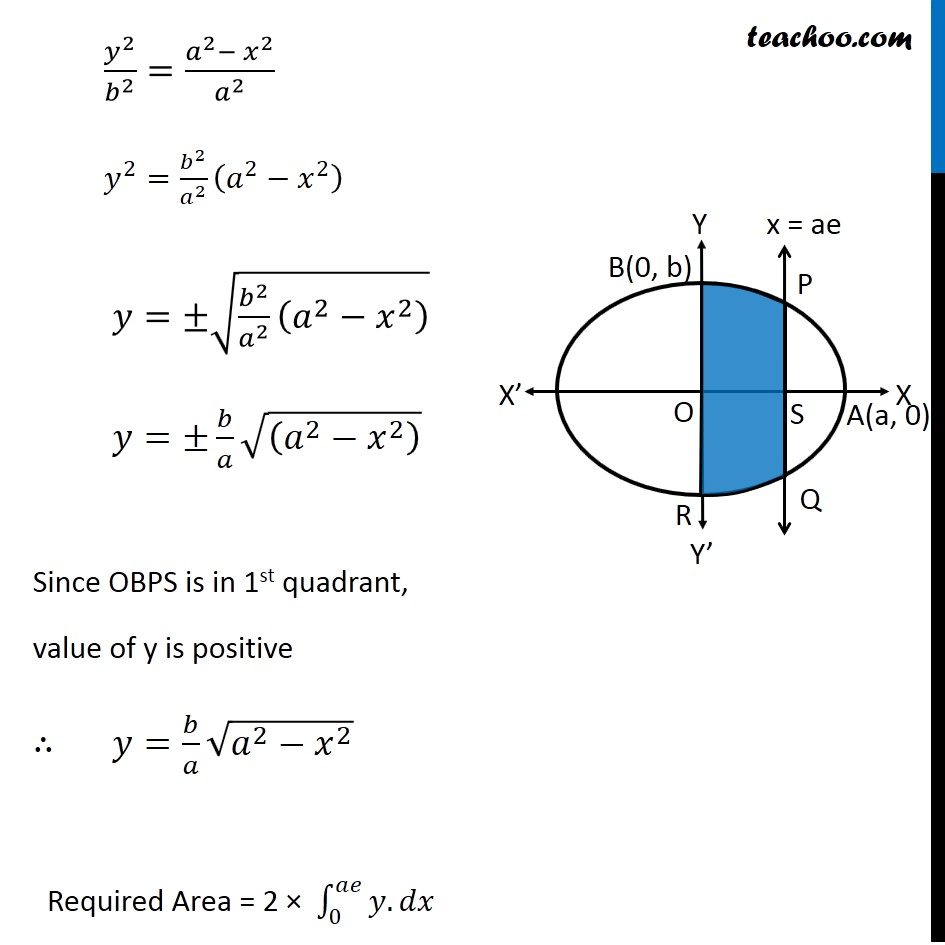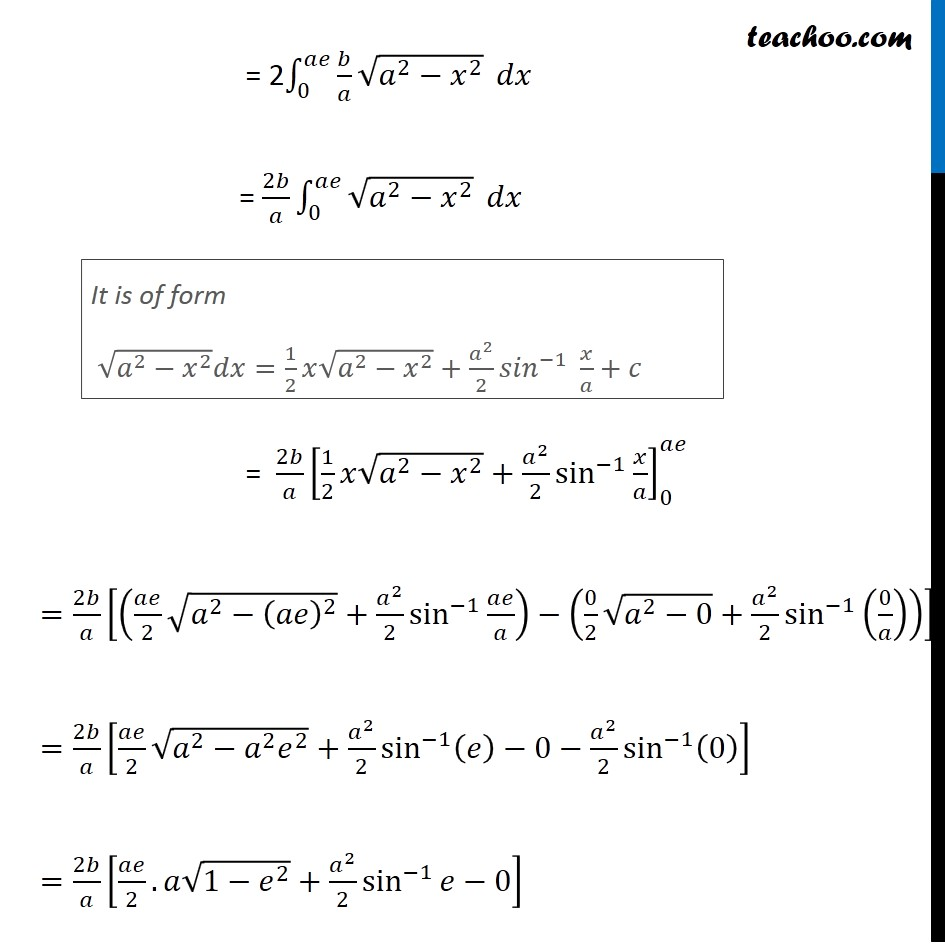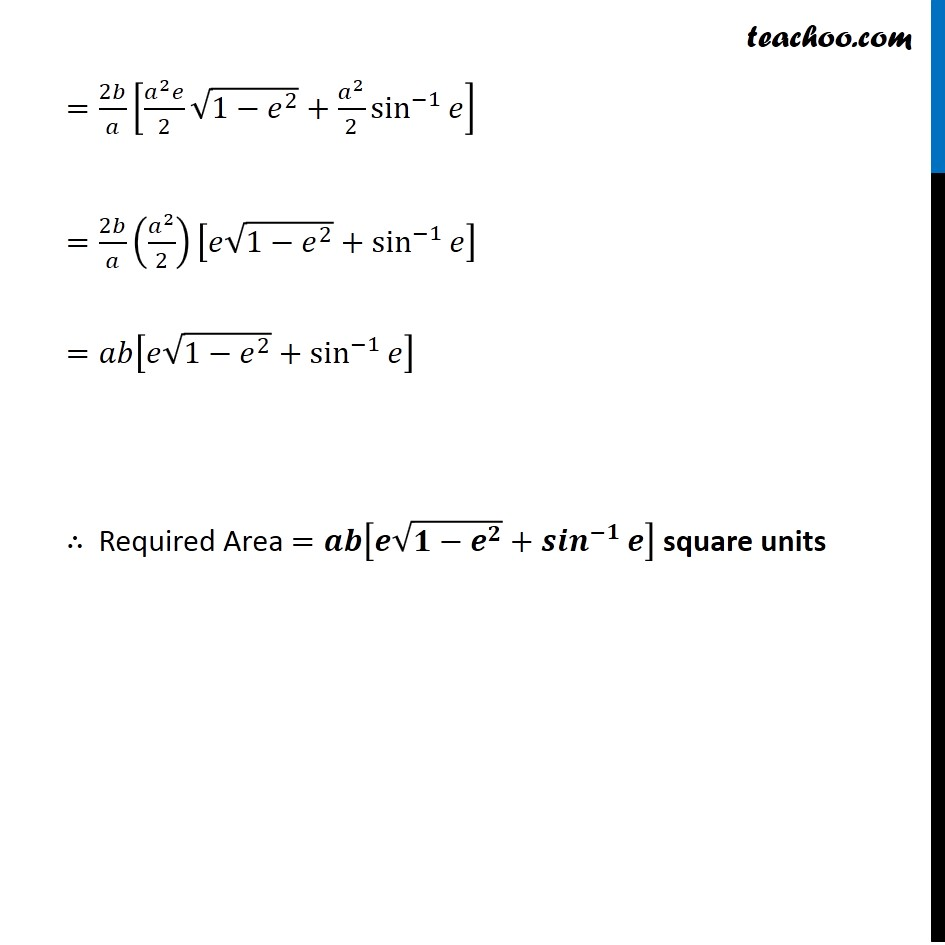Examples

Chapter 8 Class 12 Application of Integrals
Serial order wiseLearn in your speed, with individual attention - Teachoo Maths 1-on-1 Class

### Transcript

Question 3 Find the area bounded by the ellipse 𝑥^2/𝑎^2 +𝑦^2/𝑏^2 =1 and the ordinates 𝑥=0 and 𝑥=𝑎𝑒, where, 𝑏2=𝑎2 (1 – 𝑒2) and e < 1 Required Area = Area of shaded region = Area BORQSP = 2 × Area OBPS = 2 × ∫_0^𝑎𝑒▒〖𝑦.𝑑𝑥〗 We know that , 𝑥^2/𝑎^2 +𝑦^2/𝑏^2 =1 (As ellipse is symmetric about its axis ) 𝑦^2/𝑏^2 =(𝑎^2−〖 𝑥〗^2)/𝑎^2 𝑦^2=𝑏^2/𝑎^2 (𝑎^2−𝑥^2 ) 𝑦=±√(𝑏^2/𝑎^2 (𝑎^2−𝑥^2 ) ) 𝑦=±𝑏/𝑎 √((𝑎^2−𝑥^2 ) ) Since OBPS is in 1st quadrant, value of y is positive ∴ 𝑦=𝑏/𝑎 √(𝑎^2−𝑥^2 ) Required Area = 2 × ∫_0^𝑎𝑒▒〖𝑦.𝑑𝑥〗 = 2∫_0^𝑎𝑒▒〖𝑏/𝑎 √(𝑎^2−𝑥^2 )〗 𝑑𝑥 = 2𝑏/𝑎 ∫_0^𝑎𝑒▒√(𝑎^2−𝑥^2 ) 𝑑𝑥 = 2𝑏/𝑎 [1/2 𝑥√(𝑎^2−𝑥^2 )+𝑎^2/2 sin^(−1)⁡〖𝑥/𝑎〗 ]_0^𝑎𝑒 =2𝑏/𝑎 [(𝑎𝑒/2 √(𝑎^2−(𝑎𝑒)^2 )+𝑎^2/2 sin^(−1)⁡〖𝑎𝑒/𝑎〗 )−(0/2 √(𝑎^2−0)+𝑎^2/2 sin^(−1)⁡(0/𝑎) )] =2𝑏/𝑎 [𝑎𝑒/2 √(𝑎^2−𝑎^2 𝑒^2 )+𝑎^2/2 sin^(−1)⁡〖(𝑒)−0−𝑎^2/2 sin^(−1)⁡(0) 〗 ] =2𝑏/𝑎 [𝑎𝑒/2.𝑎√(1−𝑒^2 )+𝑎^2/2 sin^(−1)⁡〖𝑒−0〗 ] It is of form √(𝑎^2−𝑥^2 ) 𝑑𝑥=1/2 𝑥√(𝑎^2−𝑥^2 )+𝑎^2/2 〖𝑠𝑖𝑛〗^(−1)⁡〖 𝑥/𝑎+𝑐〗 =2𝑏/𝑎 [(𝑎^2 𝑒)/2 √(1−𝑒^2 )+𝑎^2/2 sin^(−1)⁡𝑒 ] =2𝑏/𝑎 (𝑎^2/2)[𝑒√(1−𝑒^2 )+sin^(−1)⁡𝑒 ] =𝑎𝑏[𝑒√(1−𝑒^2 )+sin^(−1)⁡𝑒 ] ∴ Required Area =𝒂𝒃[𝒆√(𝟏−𝒆^𝟐 )+〖𝒔𝒊𝒏〗^(−𝟏)⁡𝒆 ] square units